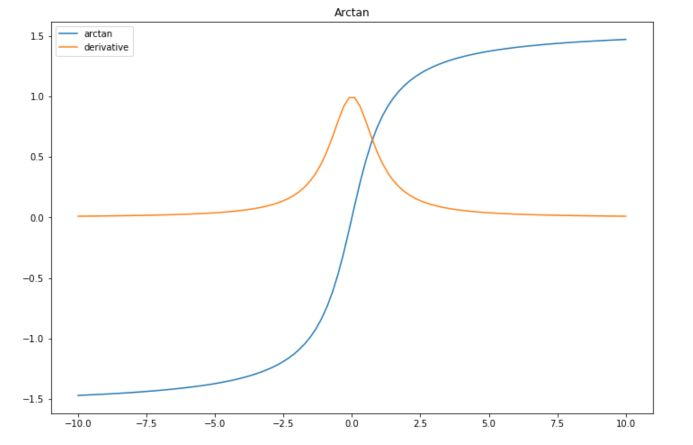When building your Deep Learning model, activation functions are an important choice to make. In this article, we’ll review the main activation functions, their implementations in Python, and advantages/disadvantages of each.

# Linear Activation

Linear activation is the simplest form of activation. In that case, $$f(x)$$ is just the identity. If you use a linear activation function the wrong way, your whole Neural Network ends up being a regression :

$\hat{y} = \sigma(h) = h = W^{(2)} h_1 = W^{(1)} W^{(2)} X = W' X$

Linear activations are only needed when you’re considering a regression problem, as a last layer. The whole idea behind the other activation functions is to create non-linearity, to be able to model highly non-linear data that cannot be solved by a simple regression !

# ReLU

ReLU stands for Rectified Linear Unit. It is a widely used activation function. The formula is simply the maximum between $$x$$ and 0 :

$f(x) = max(x, 0)$

To implement this in Python, you might simply use :

def relu(x) :
return max(x, 0)


The derivative of the ReLU is :

• $$1$$ if $$x$$ is greater than 0
• $$0$$ if $$x$$ is smaller or equal to 0
def der_relu(x):
if x <= 0 :
return 0
if x > 0 :
return 1


Let’s simulate some data and plot them to illustrate this activation function :

import numpy as np
import matplotlib.pyplot as plt

# Data which will go through activations
x = np.linspace(-10,10,100)

plt.figure(figsize=(12,8))
plt.plot(x, list(map(lambda x: relu(x),x)), label="relu")
plt.plot(x, list(map(lambda x: der_relu(x),x)), label="derivative")
plt.title("ReLU")
plt.legend()
plt.show()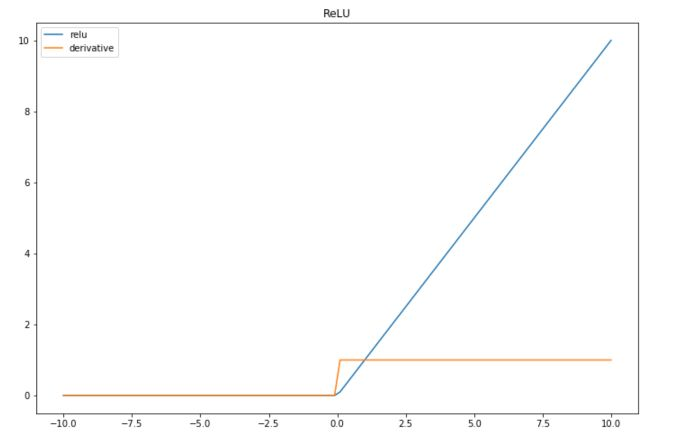Advantage Easy to implement and quick to compute Disadvantage Problematic when we have lots of negative values, since the outcome is always 0 and leads to the death of the neuron

# Leaky-ReLU

Leaky-ReLU is an improvement of the main default of the ReLU, in the sense that it can handle the negative values pretty well, but still brings non-linearity.

$f(x) = max(0.01x, x)$

The derivative is also simple to compute :

• $$1$$ if $$x>0$$
• $$0.01$$ else
def leaky_relu(x):
return max(0.01*x,x)

def der_leaky_relu(x):
if x < 0 :
return 0.01
if x >= 0 :
return 1


And we can plot the result of this activation function :

plt.figure(figsize=(12,8))
plt.plot(x, list(map(lambda x: leaky_relu(x),x)), label="leaky-relu")
plt.plot(x, list(map(lambda x: der_leaky_relu(x),x)), label="derivative")
plt.title("Leaky-ReLU")
plt.legend()
plt.show()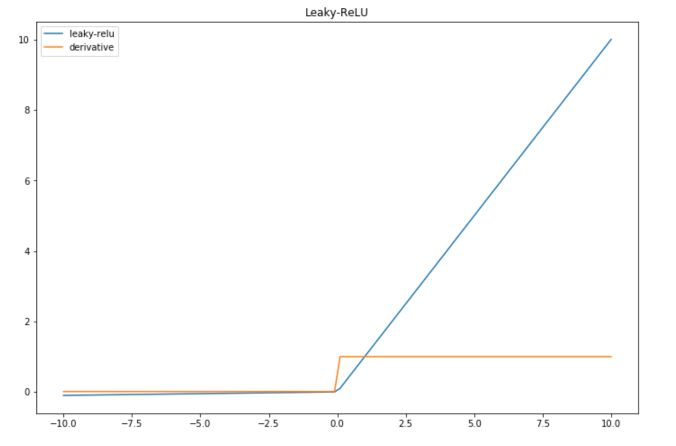Advantage Leaky-ReLU overcomes the problem of the death of the neuron linked to a zero-slope. Disadvantage The factor 0.01 is arbitraty, and can be tuned (PReLU for parametric ReLU)

# ELU

Exponential Linear Units (ELU) try to make the mean activations closer to zero, which speeds up learning.

$$f(x) = x$$ if $$x>0$$, and $$a(e^x -1)$$ otherwise, where $$a$$ is a positive constant.

The derivative is :

• $$1$$ if $$x > 0$$
• $$a * e^x$$ otherwise

If we set $$a$$ to 1 :

def elu(x):
if x > 0 :
return x
else :
return (np.exp(x)-1)

def der_elu(x):
if x > 0 :
return 1
else :
return np.exp(x)

plt.figure(figsize=(12,8))
plt.plot(x, list(map(lambda x: elu(x),x)), label="elu")
plt.plot(x, list(map(lambda x: der_elu(x),x)), label="derivative")
plt.title("ELU")
plt.legend()
plt.show()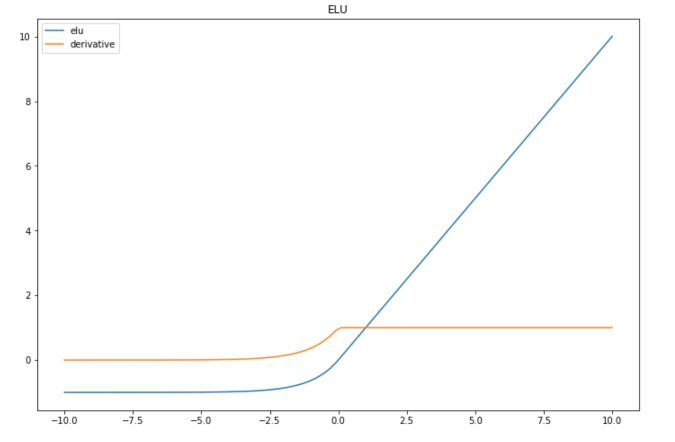Advantage Can achieve higher accuracy than ReLU Disadvantage Same as ReLU, and a needs to be tuned

# Softplus

The Softplus function is a continuous approximation of ReLU. It is given by :

$f(x) = log(1+e^x)$

The derivative of the softplus function is :

$f'(x) = \frac{1}{1+e^x}e^x$

You can implement them in Python :

def softplus(x):
return np.log(1+np.exp(x))

def der_softplus(x):
return 1/(1+np.exp(x))*np.exp(x)

plt.figure(figsize=(12,8))
plt.plot(x, list(map(lambda x: softplus(x),x)), label="softplus")
plt.plot(x, list(map(lambda x: der_softplus(x),x)), label="derivative")
plt.title("Softplus")
plt.legend()
plt.show()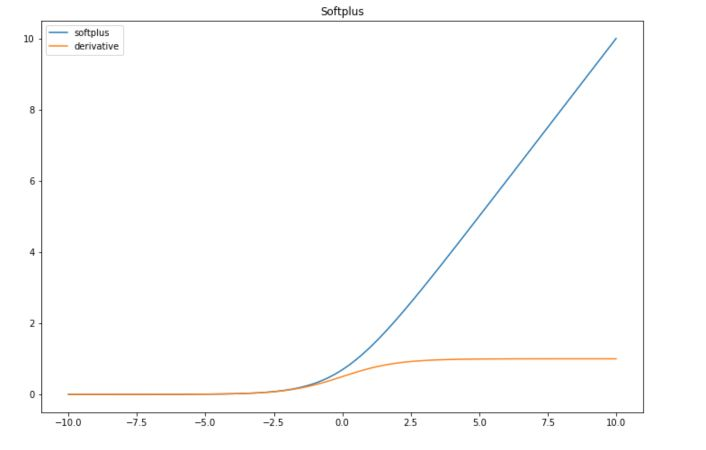Advantage Softplus is continuous and might have good properties in terms of derivability. It is interesting to use it when the values are between 0 and 1. Disadvantage As ReLU, problematic when we have lots of negative values, since the outcome gets really close to 0 and might lead to the death of the neuron

# Sigmoid

Sigmoid is one of the most common activation functions in litterature these days. The sigmoid function has the following form :

$f(x) = \frac{1}{1+e^{-x}}$

The derivative of the sigmoid is :

$f'(x) = - e^{-x} \frac{1}{(1+e^{-x})^2} = \frac {1 + e^{-x} -1}{(1+e^{-x})^2}$ $= \frac {1}{1+e^{-x}} (1-\frac {1}{1+e^{-x}}) = f(x)(1-f(x))$

In Python :

def softmax(x):
return 1/(1+np.exp(-x))

def der_softmax(x):
return 1/(1+np.exp(-x)) * (1-1/(1+np.exp(-x)))

plt.figure(figsize=(12,8))
plt.plot(x, list(map(lambda x: softmax(x),x)), label="softmax")
plt.plot(x, list(map(lambda x: der_softmax(x),x)), label="derivative")
plt.title("Softmax")
plt.legend()
plt.show()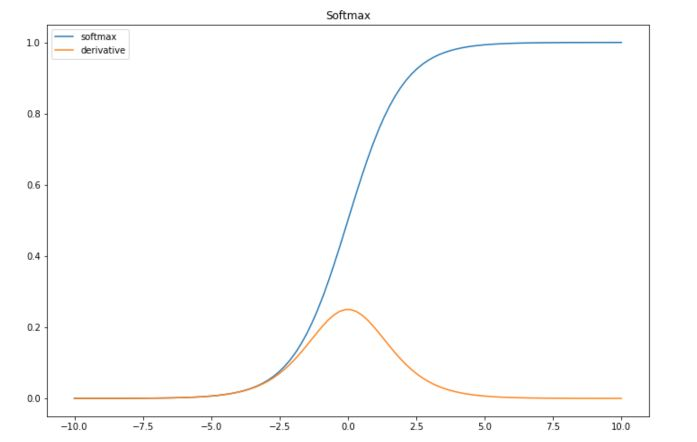It might not be obvious when considering data between $$-10$$ and $$10$$ only, but the sigmoid is subject to the vanishing gradient problem. This means that the gradient will tend to vanish as $$x$$ takes large values.

Since the gradient is the sigmoid times 1 minus the sigmoid, the gradient can be efficiently computed. If we keep track of the sigmoids, we can compute the gradient really quickly.

# Hyperbolic Tangent

Hyperbolic tangent is quite similar to the sigmoid function, expect is maps the input between -1 and 1.

$f(x) = tanh(x) = \frac {sinh(x)}{cosh(x)} = \frac {e^x - e^{-x}}{e^x + e^{-x}}$

The derivative is computed in the following way :

$f'(x) = \frac{\partial}{\partial x} \frac{sinh(x)}{cosh(x)}$ $= \frac { \frac{\partial}{\partial x} sinh(x) cosh(x) - \frac{\partial}{\partial x} cosh(x) sinh(x) } {cosh^2(x)}$ $= \frac { cosh^2(x) - sinh^2(x) } {cosh^2(x)}$ $= 1 - \frac { sinh^2(x) } { cosh^2(x) }$ $= 1 - tanh^2(x)$

Implement this in Python :

def hyperb(x):
return (np.exp(x)-np.exp(-x)) / (np.exp(x) + np.exp(-x))

def der_hyperb(x):
return 1 - ((np.exp(x)-np.exp(-x)) / (np.exp(x) + np.exp(-x)))**2


And plot it :

plt.figure(figsize=(12,8))
plt.plot(x, list(map(lambda x: hyperb(x),x)), label="hyperbolic")
plt.plot(x, list(map(lambda x: der_hyperb(x),x)), label="derivative")
plt.title("Hyperbolic")
plt.legend()
plt.show()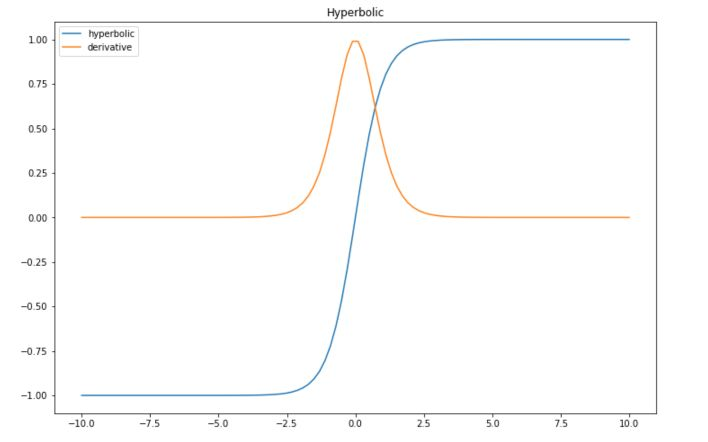Advantage Efficient since it has mean 0 in the middle layers between -1 and 1 Disadvantage Vanishing gradient too

# Arctan

This activation function maps the input values in the range $$(− \pi / 2, \pi / 2)$$. It is the inverse of a hyperbolic tangent function.

$f(x) = arctan(x)$

And the derivative :

$f'(x) = \frac {1} {1+x^2}$

In Python :

def arctan(x):
return np.arctan(x)

def der_arctan(x):
return 1 / (1+x**2)


And plot it :

plt.figure(figsize=(12,8))
plt.plot(x, list(map(lambda x: arctan(x),x)), label="arctan")
plt.plot(x, list(map(lambda x: der_arctan(x),x)), label="derivative")
plt.title("Arctan")
plt.legend()
plt.show()